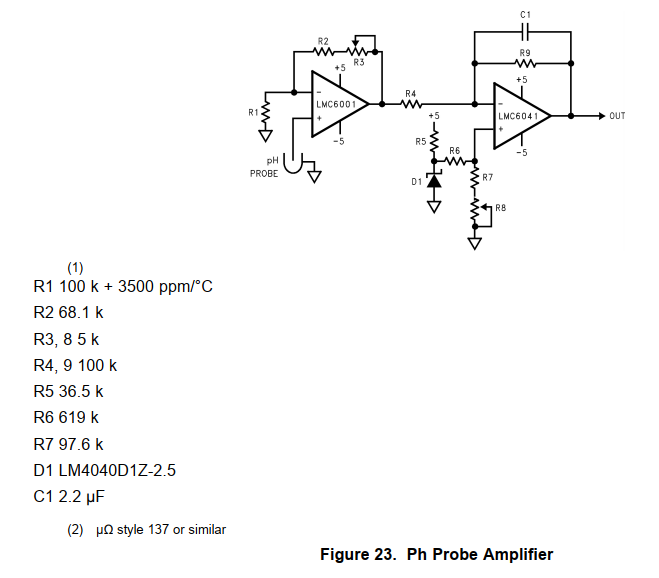If you have a related question, please click the "Ask a related question" button in the top right corner. The newly created question will be automatically linked to this question.

# LMC6001: Tell me please, where I can buy a thermal resistor R1

Part Number: LMC6001

where I can buy a thermal resistor R1 (Fig.23 of Datasheet) 100kΩ +3500ppm/°C?

Micro-ohms do not support this 137 type of resistors.is maximum 50k. So, I'm very confused (

Thanks

• Hi Alexander,

yes, it's hard to get these special resistors today.

I would change the circuit in such a way that you can use a PT1000 or even a PT10000 instead of this special resistor. The PT1000 has a temperature coefficient of +3850ppm/°C. Put a 0.1% precision metal film resistor of 10390R (10k0 + 390R) with very low temperature coefficient (< 25ppm/°C) in parallel to the PT1000. This gives an overall temperature coefficient of +3500ppm/°C.

If you take a PT10000, put a resistance of 103900R (100k + 3k9) in parallel.

Kai

• Dear Kai,

if I take even pt10000 resistor in parallel with 103900R resistance, it maybe give a result a thermal coefficient 3500/° with resulting resistance ab. 10k. But LMC6001 need 100k resistance with this TC.

Or did I misunderstand your idea?

Alexander

• Hi Alexander,

you can easily decrease the resistances R1, R2 and R3 in this circuit:The PT10000 in parallel with the 103900 resistance looks like a 9122.04 resistance. So, the resistors R2 and R3 can be decreased by a factor of 100k / 9122.04 = 10.96246. -> Take R2 = 6k2 and R3 = 500R.

Kai

• Thanks, Kai,
Its look like that the best solution is a stable 100k resistor and thermostat for cell. Because Pt10000 is even rare than type 137.
But your recommendations is the possible way to resolve a problem. Thanks.
I hope, TI take in to account this problem in next ultra low current OPA and make possible direct input of Pt1000.
A.
• Hi Alexander,

a PT1000 in parallel with a 10390R resistance should also work. Take R2 = 620R and R3 = 50R then.

Kai
• Thanks, Kai, this is very useful!